# Q Search this course CENGAGE | MINDTAP Brigham Chapter 08 End-of-Chapter Problems 0 x Question 3 of 6 Check My Wo...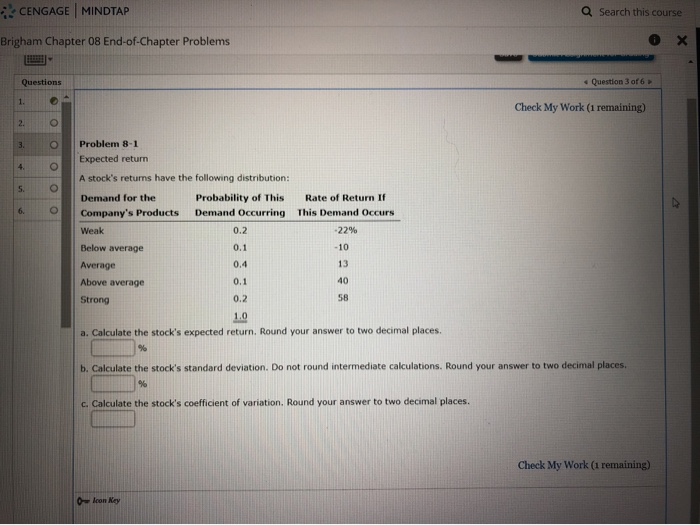Q Search this course CENGAGE | MINDTAP Brigham Chapter 08 End-of-Chapter Problems 0 x Question 3 of 6 Check My Work (1 remaining) Problem 8-1 Expected return A stock's returns have the following distribution: Demand for the Probability of this Rate of Return If Company's Products Demand Occurring This Demand Occurs Weak .22% Below average Average Above average Strong a. Calculate the stock's expected return, Round your answer to two decimal places. b. Calculate the stock's standard deviation. Do not round intermediate calculations. Round your answer to two decimal places c. Calculate the stock's coefficient of variation. Round your answer to two decimal places. Check My Work (1 remaining) 0 loon Key

Ans a - Expected Return is 15.40%

 Demand of Product Probability Rate of Returns Probability * Returns Weak 0.2 -22% -4.40% Below Average 0.1 -10% -1.00% Average 0.4 13% 5.20% Above Average 0.1 40% 4.00% Strong 0.2 58% 11.60% Expected Return 15.40%

Ans b - Standard Deviation 27.75

Demand of Product Probability Rate of Returns Probability * Returns
 X - mean
 p*(X - mean)^2
Weak 0.2 -22 -4.4 -37.4 279.752
Below Average 0.1 -10 -1 -25.4 64.516
Average 0.4 13 5.2 -2.4 2.304
Above Average 0.1 40 4 24.6 60.516
Strong 0.2 58 11.6 42.6 362.952
Expected Return 15.4 770.04
Variance 770.04
SD 27.75

SD is squire root of variance.

Ans - 3 Coefficient of variance is SD / Mean

= 27.75 / 15.40 = 1.80

Hope this helps.

##### Add Answer of: Q Search this course CENGAGE | MINDTAP Brigham Chapter 08 End-of-Chapter Problems 0 x Question 3 of 6 Check My Wo...
Similar Homework Help Questions
• ### CENGAGE MINDTAP Q Search this course A-Z Brigham Chapter 10 End-of-Chapter Problems Problem 10-8 Cost of...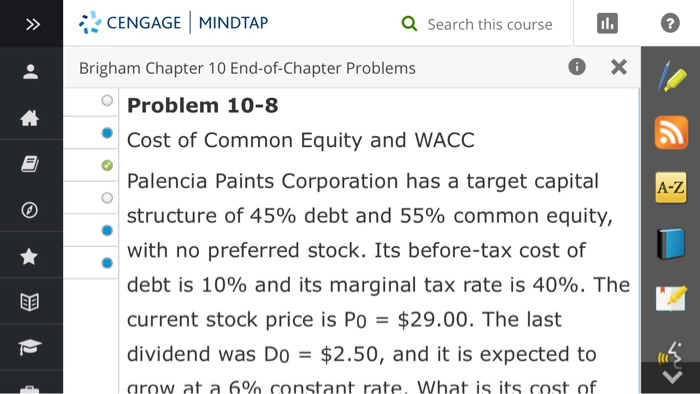CENGAGE MINDTAP Q Search this course A-Z Brigham Chapter 10 End-of-Chapter Problems Problem 10-8 Cost of Common Equity and WACC Palencia Paints Corporation has a target capital structure of 45% debt and 55% common equity, with no preferred stock. Its before-tax cost of debt is 10% and its marginal tax rate is 40%. The current stock price is Po = \$29.00. The last dividend was Do = \$2.50, and it is expected to arow at a 6% constant rate. What...

• ### We were unable to transcribe this imageCENGAGE MINDTAP Q Search this course Brigham Chapter 10 End-of-Chapter...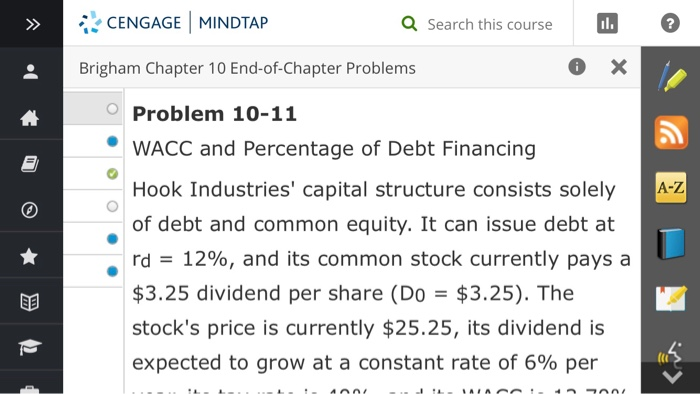We were unable to transcribe this imageCENGAGE MINDTAP Q Search this course Brigham Chapter 10 End-of-Chapter Problems expected to grow at a constant rate of 6% per year, its tax rate is 40%, and its WACC is 13.70%. What percentage of the company's capital structure consists of debt? Round your answer to two decimal places. A-Z % Check My Work (1 remaining)

• ### d Search this course Brigham Chapter 08 End-of-Chapter Problems X Check My Work (1 remaining) Problem...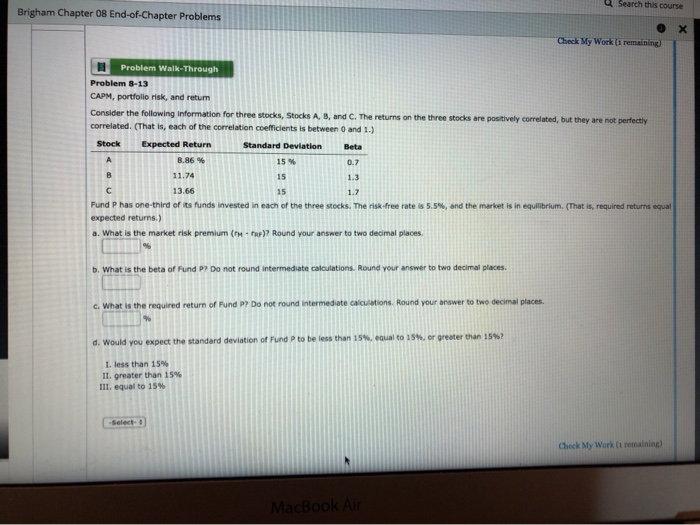d Search this course Brigham Chapter 08 End-of-Chapter Problems X Check My Work (1 remaining) Problem Walk-Through Problem 8-13 CAPM, portfolio risk, and retun Consider the following information for three stocks, Stocks A, 8, and C. The returns on the three stocks are positively correlated, but they are not perfectly correlated. (That is, each of the correlation coefficients is between 0 and 1.) Stock Expected Return Standard Deviation Beta A 8.86 % 15 % 0.7 B 11.74 15 1.3 C...

• ### Questions Problem 01 Question 3 of 11 Check My Work (3 remaining) O Click here to...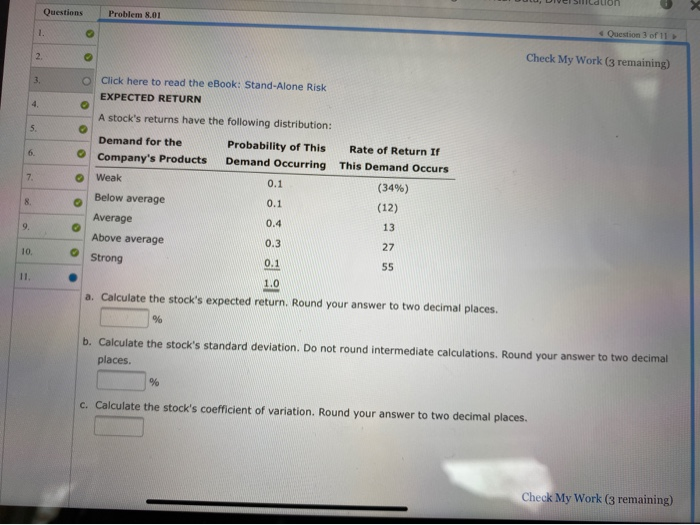Questions Problem 01 Question 3 of 11 Check My Work (3 remaining) O Click here to read the eBook: Stand-Alone Risk EXPECTED RETURN A stock's returns have the following distribution: Demand for the Probability of this Rate of Return If Company's Products Demand Occurring This Demand Occurs Weak 0.1 (34%) Below average (12) Average Above average 0.3 Strong 55 0.4 a. Calculate the stock's expected return. Round your answer to two decimal places b. Calculate the stock's standard deviation. Do...

• ### MINDTAP Q Search this course ter 09 End-of-Chapter Problems > nt: Brigham Chapter 09 End-of-Chapter Problems...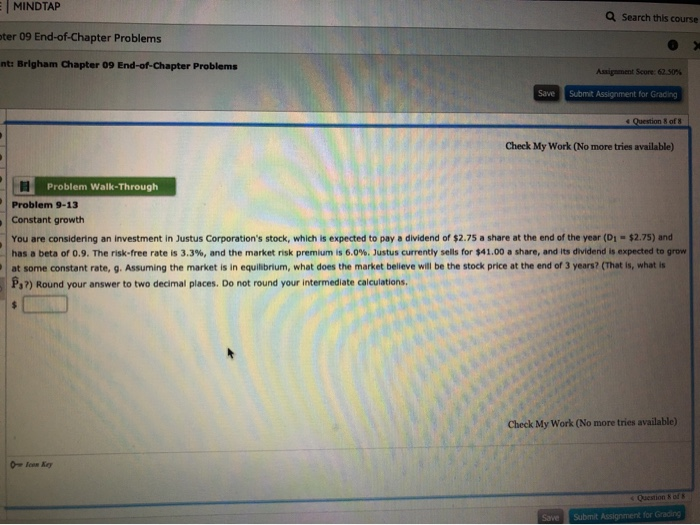MINDTAP Q Search this course ter 09 End-of-Chapter Problems > nt: Brigham Chapter 09 End-of-Chapter Problems Assignment Score: 62.50% Save Submit Assignment for Grading Question of Check My Work (No more tries available) H Problem Walk-Through Problem 9-13 Constant growth You are considering an investment in Justus Corporation's stock, which is expected to pay a dividend of \$2.75 a share at the end of the year (D1-\$2.75) and has a beta of 0.9. The risk-free rate is 3.3%, and the...

• ### Questions Check My 10. Problem 8-1 Expected return A stock's returns have the following distribution: Demand for th...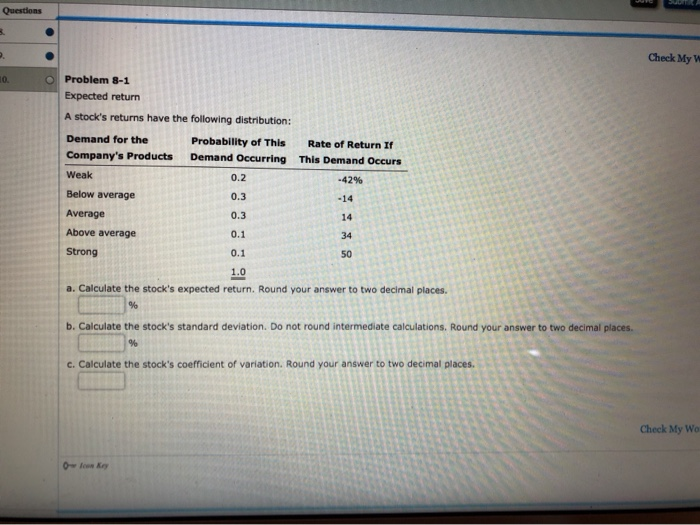Questions Check My 10. Problem 8-1 Expected return A stock's returns have the following distribution: Demand for the Probability of This Company's Products Demand Occurring Weak 0.2 Below average Average 0.3 Above average 0.1 Rate of Return If This Demand Occurs -42% Strong a. Calculate the stock's expected return. Round your answer to two decimal places. b. Calculate the stock's standard deviation. Do not round intermediate calculations. Round your answer to two decimal places c. Calculate the stock's coefficient of...

• ### why is this wrong? Check My Work (3 remaining) eBook 1 Problem Walk-Through A stock's returns...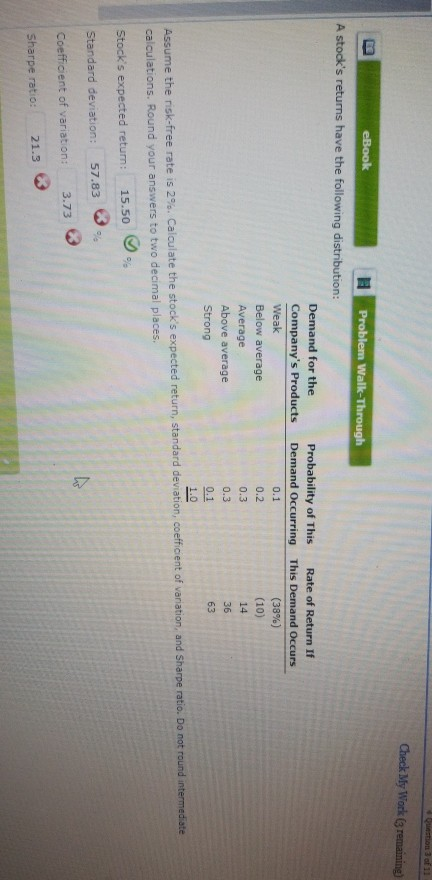why is this wrong? Check My Work (3 remaining) eBook 1 Problem Walk-Through A stock's returns have the following distribution: Demand for the Probability of This Company's Products Demand Occurring Weak Below average Average 0.3 Above average 0.3 Strong Rate of Return If This Demand Occurs (38%) (10) 14 0.2 Assume the risk-free rate is 2% Calculate the stock's expected return, standard deviation coefficient of variation and Sharpe ratio. Do not found intermediate calculations. Round your answers to two decimal...

• ### Problem 8.01 (Expected Return) Question 1 Check My Work (No more tries availal eBook A stock's...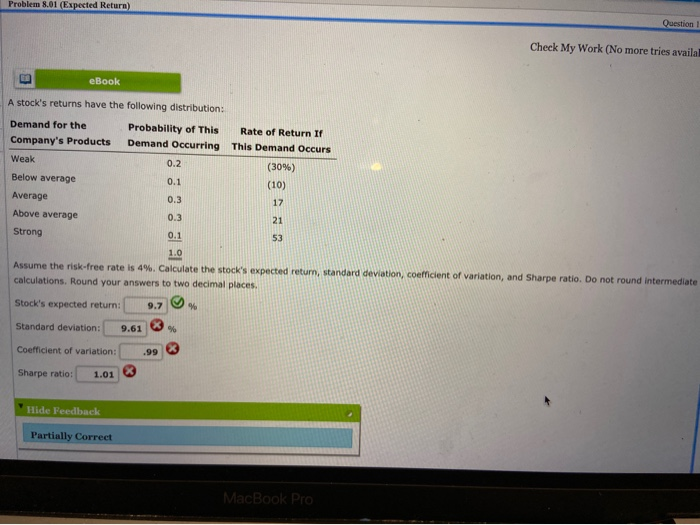Problem 8.01 (Expected Return) Question 1 Check My Work (No more tries availal eBook A stock's returns have the following distribution: Demand for the Probability of This Rate of Return If Company's Products Demand Occurring This Demand Occurs Weak (30%) (10) Below average Average Above average Strong Assume the risk-free rate is 4% Calculate the stock's expected return, standard deviation coefficient of variation, and Sharpe ratio. Do not round Intermediate calculations. Round your answers to two decimal places. Stock's expected...

• ### oymentid-56063033453463044987234693 8elSBN =9781 3372992208id-531963581 8snapshotld=1265231& MINDTAP Search this c ter 09 End-of-Chapter Problems t: Brigham Chapter...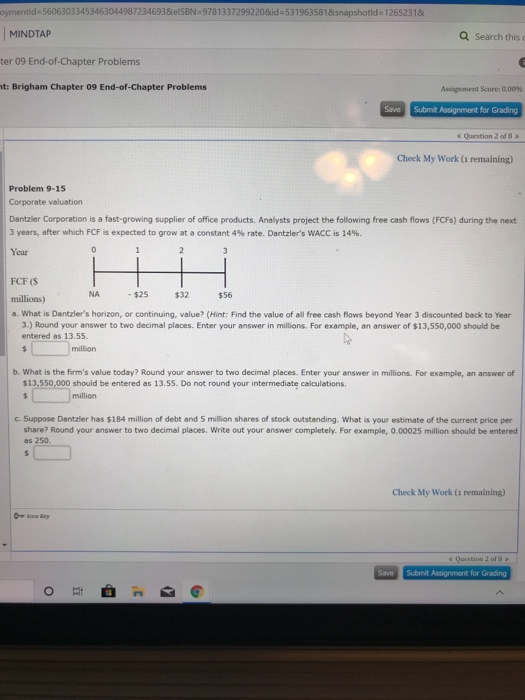oymentid-56063033453463044987234693 8elSBN =9781 3372992208id-531963581 8snapshotld=1265231& MINDTAP Search this c ter 09 End-of-Chapter Problems t: Brigham Chapter 09 End-of-Chapter Problems Assignment Score: 0.00% Submit Assignment for Grading Save Question 2 of 8 Check My Work (1 remaining) Problem 9-15 Corporate valuation Dantzler Corporation is a fast-growing supplier of office products. Analysts project the following free cash flows (FCFs) during the next 3 years, after which FCF is expected to grow at a constant 4 % rate. Dantzler's WACC is 14 %...

• ### MINDTAP Search this course From Cengage Chapter 2 Assignment Check My Work 2. 3 4 3....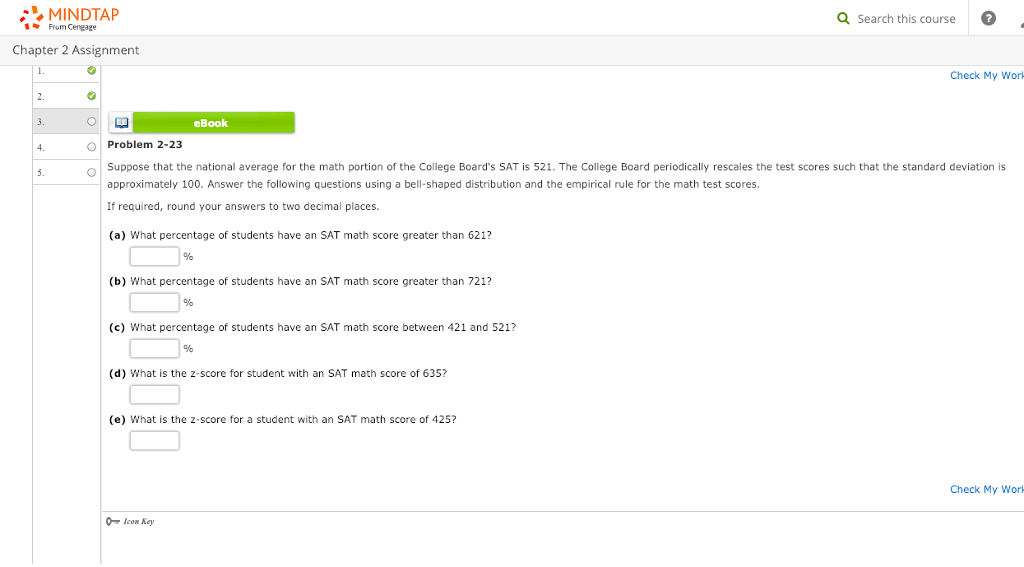MINDTAP Search this course From Cengage Chapter 2 Assignment Check My Work 2. 3 4 3. eBook Problem 2-23 approximately 100. Answer the following questions using a bell-shaped distribution and the empirical rule for the math test scores If required, round your answers to two decimal places. (a) What percentage of students have an SAT math score greater than 621? o Suppose that the national average for the math portion of the College Board's SAT is 521The Cllege Board periodically...

Need Online Homework Help?